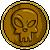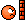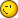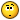# Cas.solve

4 replies to this topic

### #1Colombia

Colombia

•• Members
•• 94 posts
• Gender:Male
• Location:Venezuela

• Calculators:
algebra FX 2.0 plus

Posted 11 May 2007 - 10:38 PM

Hey to everybody

I need some help with this code:

require("cas")
local y,z
y={"x+y=3","y^2=4"}
z=cas.solve(y,"{x,y}")
print(z)However, the next code works very well

require("cas")
local y
y=cas.solve({"x+y=3","y^2=4"},"{x,y}")
print(y)

If anyone knows why I can?t put ,just, the variable into the solve function? I will apreciated.

### #2Orwell

Orwell

Casio Overlord

•• Members
•• 777 posts
• Gender:Male
• Location:Paris - France

• Calculators:
Casio AFX 1.02 / Casio ClassPad 300

Posted 12 May 2007 - 07:48 PM

Actually the correct code is

```require("cas")
local y
y=cas.solve("{x+y=3, y^2=4}","{x,y}")
print(y)```
It's completely different than what you wroteYou have to remember something : the CAS only understands numbers, strings, and CAS expressions.
You cannot pass a Lua table to a CAS function.

"{x+y=3,y^2=4}" is a string, you can give it as argument.
But {"x+y=3","y^2=4"} is a Lua table with two strings, and the CAS does not understand it.

If you really want to give a list with 2 expressions as strings, write something like
```exp1 = "x+y=3"
exp2 = "y^2=4"
y=cas.solve( "{" .. exp1 .. "," .. exp2 .. "}", "{x,y}")```
and NOT
`y=cas.solve( {exp1, exp2}, "{x,y}")`
which is incorrect.

When you gives a number or a CAS expression as parameter of a CAS function, it is actually transformed into a string. But tables aren't converted in a comprehensible way for the CAS (by default; this can be changed).### #3Orwell

Orwell

Casio Overlord

•• Members
•• 777 posts
• Gender:Male
• Location:Paris - France

• Calculators:
Casio AFX 1.02 / Casio ClassPad 300

Posted 12 May 2007 - 08:26 PM

When you gives a number or a CAS expression as parameter of a CAS function, it is actually transformed into a string. But tables aren't converted in a comprehensible way for the CAS (by default; this can be changed).For those who could be wondering, here is a trick to do that:

The idea is to change the way the tables are converted to strings (for example when you print it or when you use it in a CAS expression). We can define a function to do that, by changing the lists' metatables:

```function caslist(list)
meta = getmetatable(list) or {}
meta.__tostring=function()
local s = "{"
local notfirst = false
for _,v in ipairs(list) do
if notfirst
then s = s.."," end
s = s .. v
notfirst = true
end
return s .. "}"
end
setmetatable(list,meta)
return list
end```
With this you can create and use lists like this:

```exp1 = "x+y=3"
exp2 = "y^2=4"
y=cas.solve( caslist{exp1, exp2}, "{x,y}")```

Note: remember that with CPLua,
`cas.foo(arg1,arg2,...)`
is just syntactic sugar for
`cas("foo(" .. tostring(arg1) .. "," .. tostring(arg2) ..   ...  .. ")")`### #4Colombia

Colombia

•• Members
•• 94 posts
• Gender:Male
• Location:Venezuela

• Calculators:
algebra FX 2.0 plus

Posted 12 May 2007 - 10:46 PM

Thanks Orwell.
I understood all

### #5Orwell

Orwell

Casio Overlord

•• Members
•• 777 posts
• Gender:Male
• Location:Paris - France

• Calculators:
Casio AFX 1.02 / Casio ClassPad 300

Posted 13 May 2007 - 07:58 AM

You're welcomeJust a word about my second post: unfortunately it does not seem to work as it shouldI've found where the problem comes from, it should be fixed in th next version#### 0 user(s) are reading this topic

0 members, 0 guests, 0 anonymous users